# Einstein, Pythagorean, E=MC Squared, and the String Theory of Everything

MY ESOTERIC likes to think of himself as a bit of a polymath with degrees in Statistics, Accounting, Computer Science, & Operations Research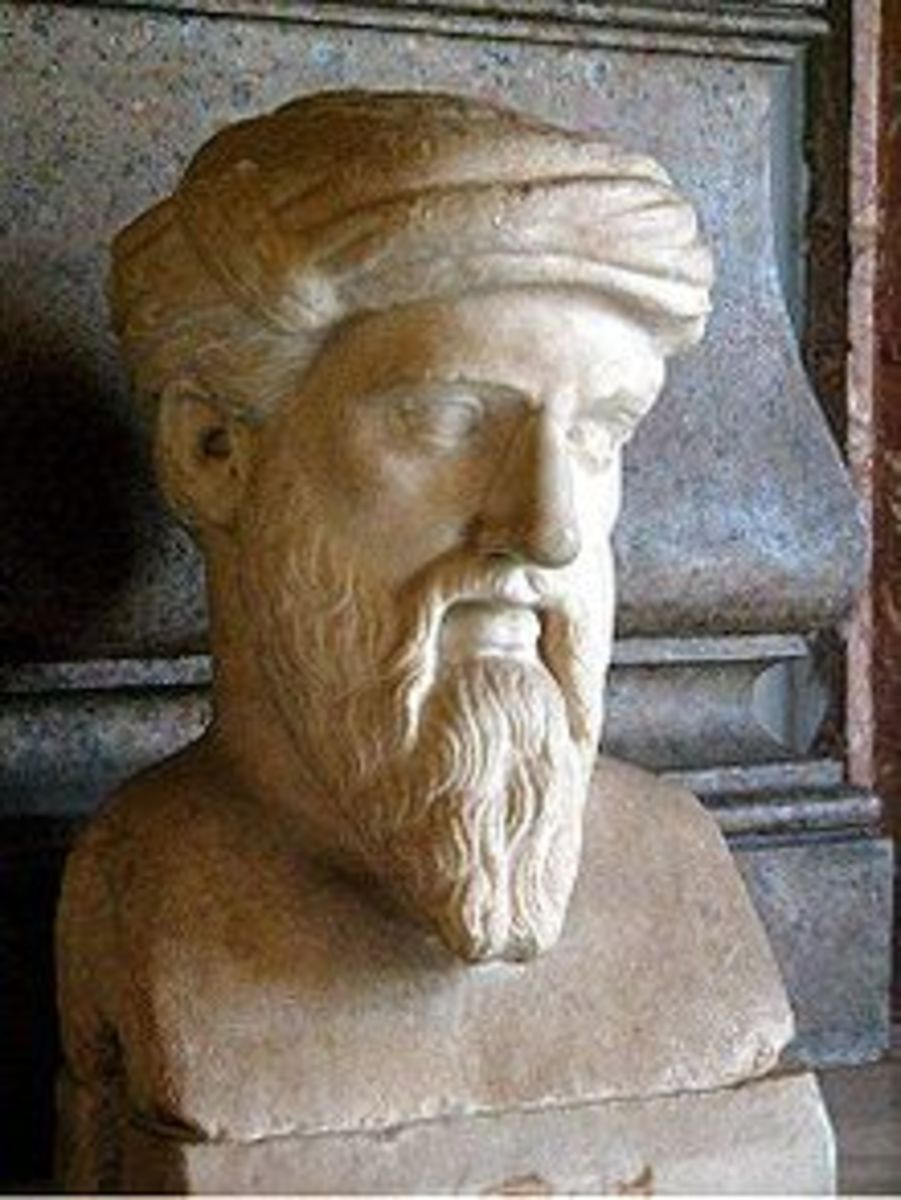PYTHAGORAS () of SAMOS 570 BC - 495 BCWikipedia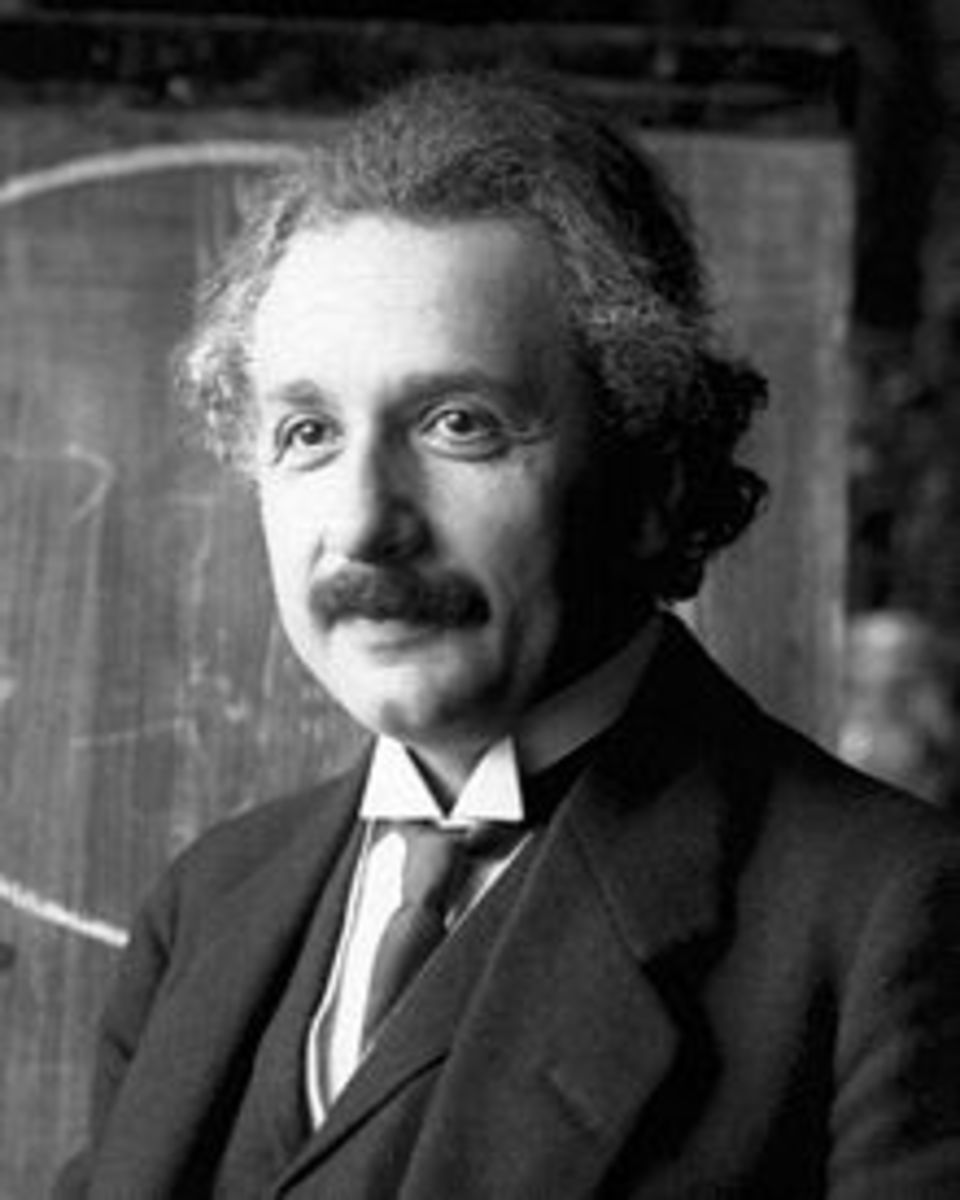ALBERT EINSTEIN in 1921: 1879 - 1955Wikipedia

## A Simple Little Challenge

I thought I would take a break from my normal topics and begin an article in another area that has always held great fascination for me: science. As I have mentioned in my profile and other places, Science aka Natural Philosophy, plays a major role in my overall philosophical beliefs. For example, I think science holds the key to understanding Free Will, but, that is not the purpose of this article.

What I would like to do in a few short sections is:

1. introduce why Pythagorean's Theorem works the way it does (you remember this one don't you; Hypotenuses, sum of squares, and all that? If not. patience) and
2. derive, in layman's terms, Albert Einstein's famous equation, E = MC2. Shouldn't be too hard, don't you think?

How did this project come about? On a road trip from Hot Springs, AR back to my home in Florida. When I take these trips I entertain myself by listening to lectures on various subjects of interest; for me, this is often music to my ears, and since I drive by myself, nobody else has to suffer my strange affliction. Anyway, on this trip, I played a lecture title "Superstring Theory: The DNA of Reality " by Professor S. James Gates, Jr., the University of Maryland at College Park. In the course of this lecture, Professor Gates uses the Pythagorean Theorem in many of his descriptions on String Theory, so, he laid out the foundation behind the theorem in a way I have never seen before and in doing so made something that was basically opaque to me, clear. At the same time, he stated you could use the principals of this ancient theorem to derive Einstein's famous equation that relates energy and matter, E = MC2

## Pythagorean Theorem: Simplest Form in 2-Dimensions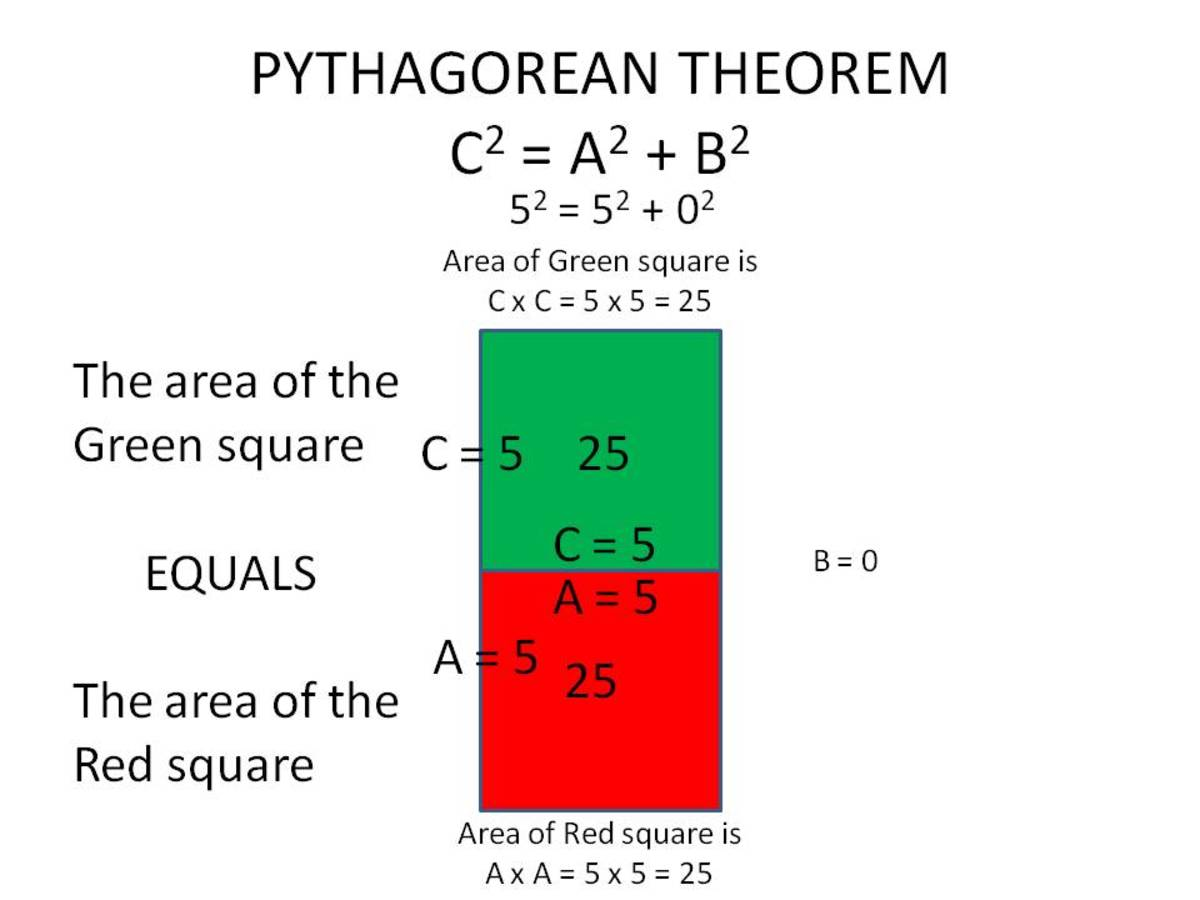PYTHAGOREAN THEOREM C=5. A=5. B=0 CHART 1My Esoteric

## Pythagorean Theorem

WHAT I am about to show is probably well known to many but was brand new me; this shows you how much I paid attention in college and I was a math major to boot, lol; rote is a wonderful thing. OK, for those who don't recognize Pythagorean's Theorem yet, it is the theorem that says:

"The square of the length of the hypotenuse (c) of a right triangle is equal to the sum of the square of each leg (a, b) of the triangle " or C2 = A2 + B2

As an aside, it is interesting to note that Pythagoras of Samos (570 BC - 495 BC) is not who actually derived this equation, it was well known by the Babylonians 1,000 years earlier.

I suspect my high school instructors tried to teach me why this equation worked but, if they did, it never sunk in. All I ever knew was the formula, when and how to apply it. Well, to understand how we get from C2 = A2 + B2 to E = MC2 we need to actually know why Pythagorean's Theorem really works; so, here goes.

If you look at Chart 1, you will see I drew two squares of equal size; in this case all sides are 5. That means, of course, that the Area of each square must be 25. Now, as you can also see that I stacked the two squares on top of each other so that they have one side in common; that side is the base of one square and the top of the other. From this, it is easy to see that the Areas of the two squares are and must be the same.

Now, what is a right triangle? It is simply a triangle which has the property that one of its angles is exactly 90 degrees; nothing more, nothing less. Since a triangle, by definition, is made of three sides and three angles, we can label these sides A, B, and C; and angles < a, < b, < c, respectively. By convention, the hypotenuse, the side opposite the 90-degree angle is labeled C.

In our first example, Chart 1, something is missing, side 'B'; it is shown with length zero. Even though this picture looks like two squares stacked on top of each other, it really is a Right Triangle. How, you ask? Simple, I say. One of the three angles is zero degrees leading to the side opposite (B) being length zero.

Since this is really a right triangle, Pythagorean's Theorem applies. Consequently, you should be able to see what the equation is actually saying is that the area of the square attached to the hypotenuse (C) is equal to the sum of the area of the squares attached to the lines opposite the other two angles of the triangle. In this first case, since one of the angles is zero, the side that would be opposite of that angle is non-existent and we are left with the stacked squares.

In Chart 2, you see we raised one corner of the Green square a bit while maintaining the length of side 'C' so that the area of the square does not change. Well, when we do this, two things happen: side 'A' of the Red square gets shorter and we create side 'B' of a new square, the Blue square; remember, we are dealing with a right triangle here. What is happening here? We are maintaining equality, that is what.

Scroll to Continue

## Read More From Owlcation

Because we are dealing with a closed system, the Green and Red squares comprise the total system and they must be equal in all dimensions because they are squares and share a common side, the initial equality must be maintained. Just because we change the position of one of the squares, so long as we retain the integrity of the right triangle, we don't invalidate the relationship.

So, as we lift the Green square we create a recognizable right triangle, but, in doing so we shrunk the Red square, in our example for 5 units to 4 units. Given side 'A' is now 4, that means the area of the Red square is 16 which is now less than the Green square. This means, of course, that we need to bring the total area of the non-Green squares back up to 25. This is accomplished with the creation of the new leg 'B' and the Blue square. As you can see, the Blue square requires an area of 9 so that with the Red square we still have a total area of 25.

No matter how little or how much you raise the Green square, this must be true. In order to maintain the equality within this closed system, you will have to add enough area to the Blue square such that, when combined with the Red square, it equals the area of the Green square.

To bring us back from the areas of the squares to the length of the legs of a right triangle all you need to note is that the area of any one of those squares is exactly one of its sides multiplied by itself or, said another way, one of its sides squared.

## Pythagorean Theorem in 3-Dimensions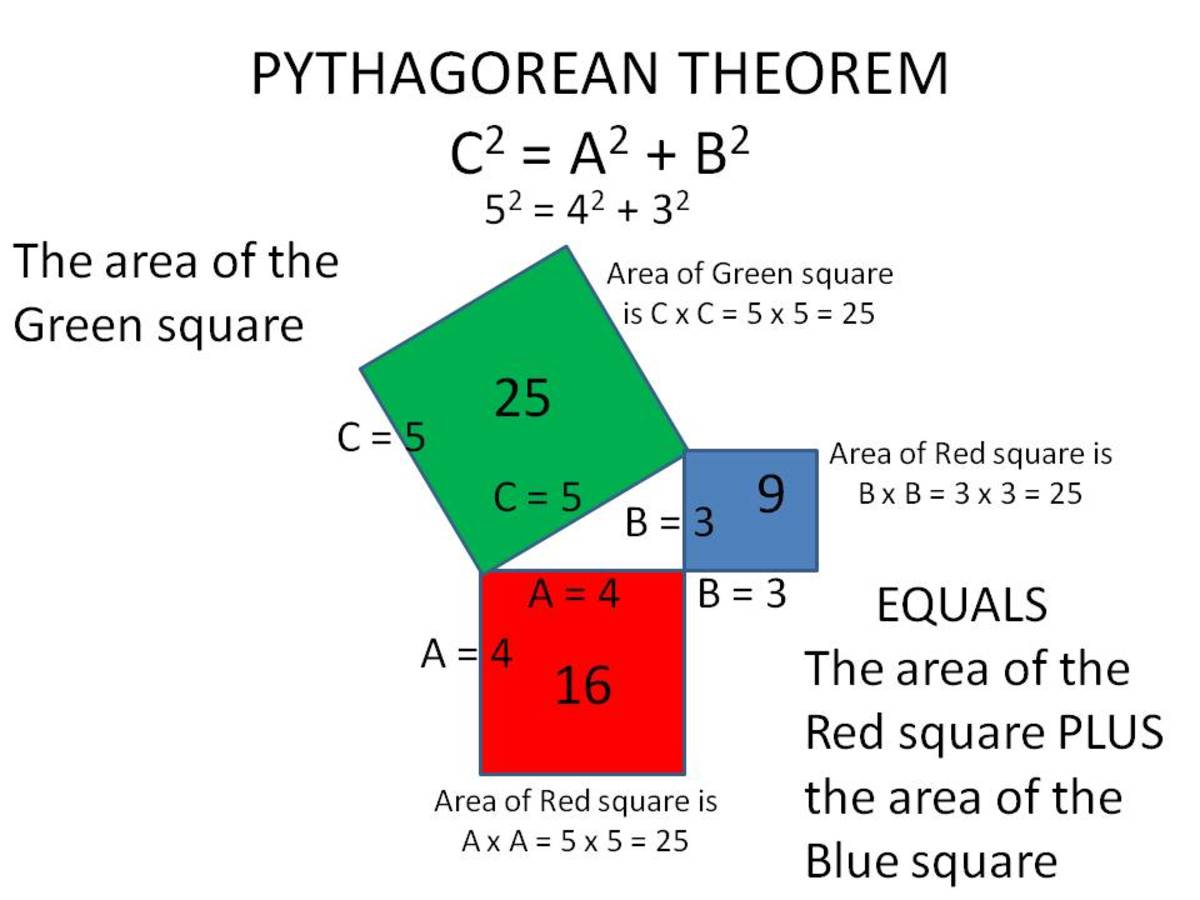PYTHAGOREAN THEOREM C=5, A=4, B=3 CHART 2My Esoteric

## Expanding Our View

Pythagorean's Theorem, as we normally understand it, works in two dimensions; some paired combination of length, width, or height where any two of these dimensions correspond to the 'A' and 'B' legs of the right triangle. Without going into any proof, let me state the obvious, Pythagorean's Theorem also works in three dimensions, length (L), width (W), and height (H). There is nothing tricky about the new formula, it is simply adding one more term to the old formula. For reasons which will become apparent shortly, I am going to replace the 'A' and 'B' in the equation with either 'L', 'W'. or 'H' while leaving the hypotenuse the same, 'C'.

So, assume first we are dealing with length and width, then we have C2 = L2 + W2 for our two-dimensional world. If we want to talk in terms of all three dimensions, we get, C2 = L2 + W2 + H2. As it turns out, this same expansion can be used regardless of the number of dimensions we want to talk about; all you do keep adding squared terms. For our purposes, however, we are only going to add one more which I will call 'T' so that my new "Pythagorean's Theorem" will read C2 = L2 + W2 + H2 + T2.

## Pythagorean Theorem in 4-Dimensions with Units of Measure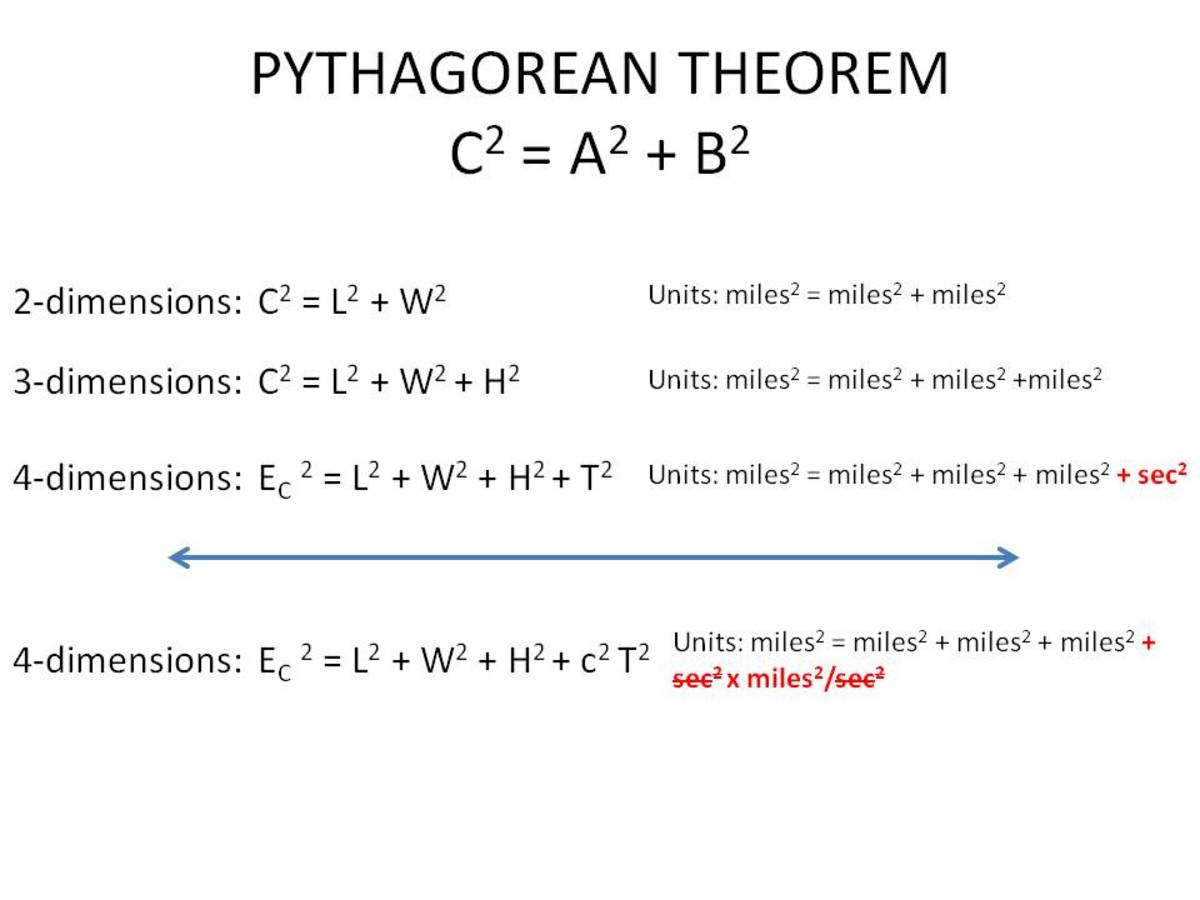ADDING TIME and UNITS TO PYTHAGOREAN'S THEOREM CHART 3My Esoteric

## Einstein's Hypotenuse

WHAT IS this 'T' dimension? Well, remember who we are talking about here, Einstein. What is one of the things Einstein is most famous for? Proving to the world that the passage of Time is not constant but can change. In other words, the passage of 10 seconds as seen by me, may be the passage of 20 seconds as seen by you. The upshot of Albert Einstein's science is that
Time is a dimension no different than length, width, and height; time is simply a fourth dimension and is the 'T' in our expanded Pythagorean Theorem.

With the addition of 'T' dimension, some have started calling the resulting hypotenuse of our four-dimensional right triangle the "Einstein Hypotenuse EC."

I will try to stay as far away from mathematics as possible so that there is at least a modicum of a chance I won't lose my non-math oriented readers but nevertheless some will be necessary.

The first complicating factor we must introduce is that of units. So far in the charts I presented, I used simple numbers with no real representation of what they stood for. Most probably, you took them to mean distances of some sort, but I never really said until I changed the labels for 'A' and 'B' to 'L,' etc. Now, however, I do mean distances, and, since I am writing to a mostly American audience, although I must tip my hat to the many Canadians that follow me as well, I will use miles as my distance measure, although it really doesn't matter. For time, I will use the normal unit of seconds.

This immediately presents a problem because, as you can see from Chart 3, we are mixing "miles" and "seconds"; mathematically, you can't do that. As a result, we need to start doing "math magic"; it is also, as it turns out, the first step in turning a "sow's ear into a silk purse."

OK, what is the problem? We have "miles" squared equal to three times "miles" squared plus "seconds" squared; we got to do something about those seconds. What we must find is a constant which relates distance with time and, guess what, we have one, provided by none other than Mr. Einstein ... light or rather the Speed of Light, 'c.' According to Einstein, the speed of light is a constant, some 186,282 miles/sec, so it does not fundamentally disturb anything by multiplying the Time dimension by this constant. But, it does simply things for us a bit because the units of 'c' is miles/sec so, when c is multiplied by Time all you have left, in terms of units, is miles or, in our situation, miles squared. As a result, this "time" term is now in the same units as the rest of the equation and the equation is in balance.

Therefore. referring to Chart 3, we have Einstein's Hypotenuse, EC2 = L2 + W2 + H2 + c2T2, where the units are in terms of length. Even the time dimension is in terms of length because we multiplied time by the speed of light, a constant.

(Note: Einstein did one more thing to adapt the Pythagorean Theorem to his Theory of Special Relativity, he changed the signs on the length terms from positive to negative so that the equation actually reads EC2 = c2T2 -L2 - W2 - H2. Why he did this is beyond my understanding at the moment, but the fundamentals behind the Pythagorean Theorem do not change. For my purposes, as you will see, the negative signs do not matter so I will leave the equation alone.)

## Einstein's Genius: Representing Momentum and Energy in terms of Pythagorean's Theorem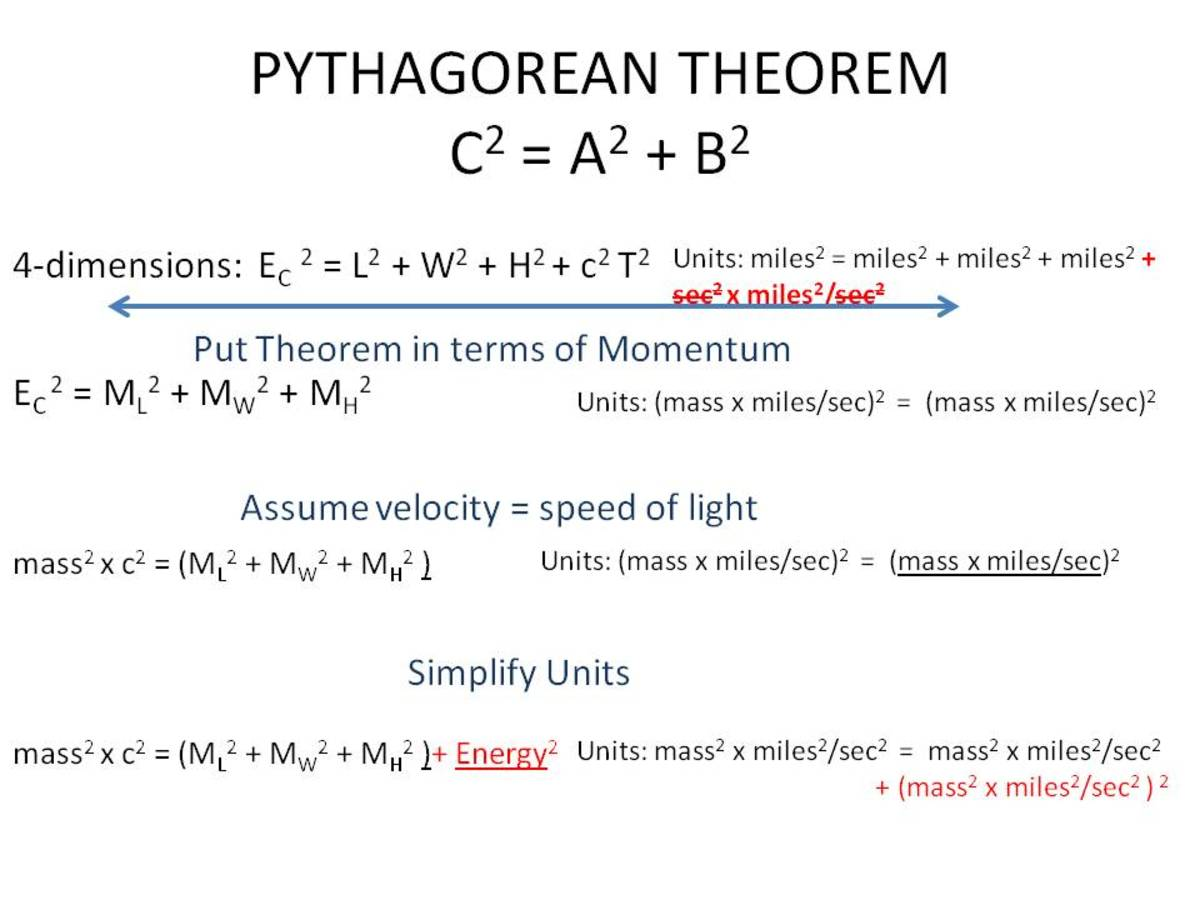HOW MOMENTUM AND ENERGY CAN BE RELATED CHART 4My Esoteric

## Getting to E = MC Squared

AS YOU have seen, Pythagorean's Theorem is used to talk about distances, inches, feet, miles, etc. Even so, it was Einsteins genius that saw how it could also be used relative to Momentum and Energy. For those who don't know, Momentum is the Mass of an object times its Velocity while Energy, the ability of a system to do work, is a constant times Mass times Velocity2. Notice also that Velocity is a Distance divided by time. Since both Momentum and Energy are, so to speak, a function of Distance, they can, with the proper mathematical manipulations, be thought of as Areas such as we have in our original formulation of Pythagorean's Theorem. These units are noted in Chart 4 and, when you only consider Pythagorean's Theorem in terms of momentum, then it is easy to see the area of the hypotenuse squared is (Mass x Distance/Time)2

Mathematics allows you to multiply both sides of an equation by a constant without changing the nature of the equation. So, if we do that here and multiply each side by the speed of light squared, which has the same units as the existing terms, specifically (distance/time)2. Consequently, as you can see in Chart 4 we can express the left-side of the Pythagorean Theorem as mass2 x c2 or m2c2.

Let's add in, now, the 4th dimension of Energy, where the first three dimensions are momentum in the up-down, left-right, and back-forth directions. The problem with Energy is its terms, mass x distance2/time2. This must be corrected and can be done so by dividing by the speed of light 'c' which gives (mass x distance/time)/c.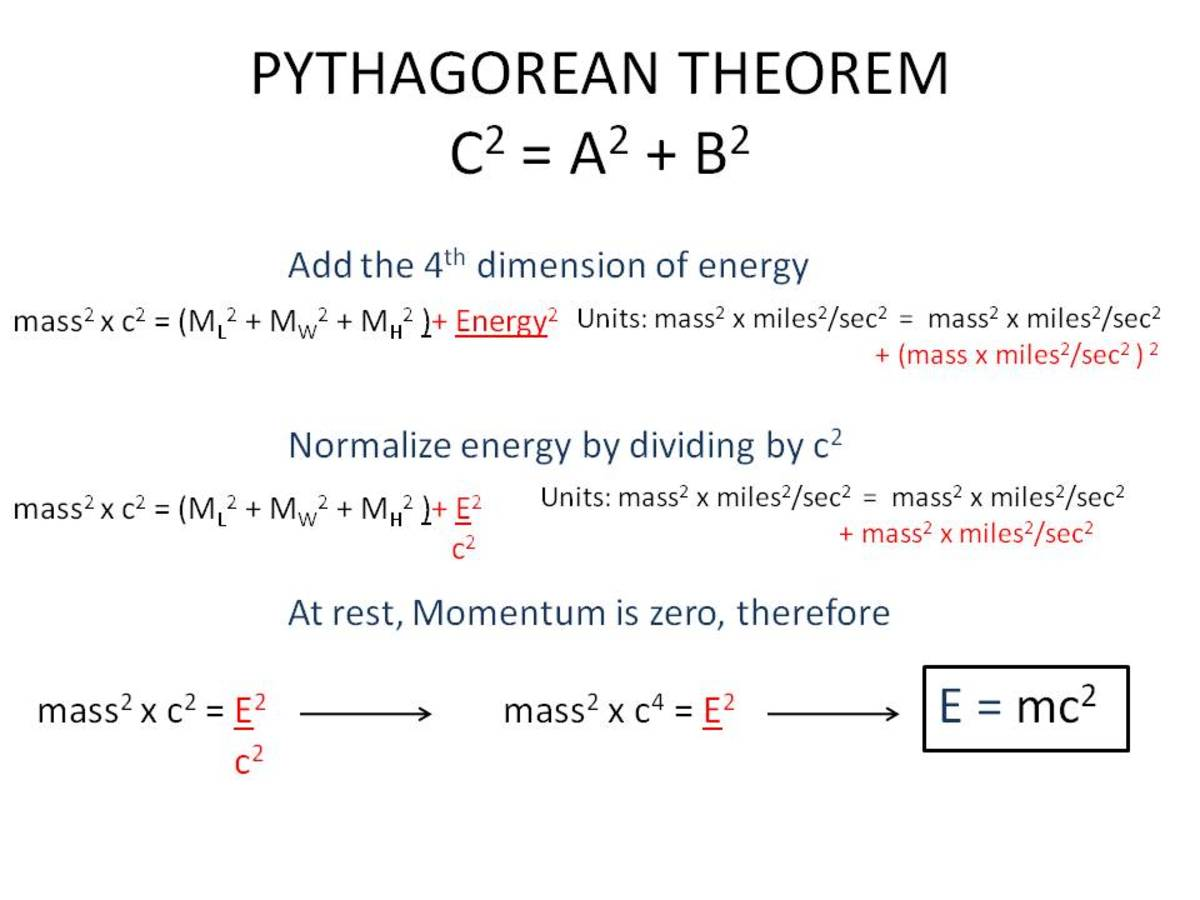GETTING TO E = MC SQUARED CHART 5My Esoteric

So, substituting back into E2, we get ((mass x distance/time)/c)2 or mass2 x (distance/time)2/c2.which looks exactly like the left-hand term we previously developed. Chart 5 shows this.

One more assumption is now required, assuming that the system we are talking about is at rest then an interesting thing happens. Objects with zero velocity have zero momentum, therefore, all the Momentum terms in EInsteing's Hypotenuse equation become zero.

From here it is a simple matter to finish our work. From Chart 5, we see that (mass2 x (distance/time)2 is equal to E2 so we have E2/c2. To put it all together and flipping sides, we get E2/c2 = m2c2. Multiplying each side by c2 you get E2 = m2c4. Taking the square root of each side and guess what, one of the most famous equations in the world emerges

• E = mc2 Voila! Who needs the Calculus, algebra is just fine thank you.

(To you real mathematicians out there, be kind in your comments if you would. It has been a decade or so since I delved this deep. which I realize is still just the surface, into the mechanics of algebra and units. Let me know if I made any logical errors in getting from the two knowns, Pythagorean's Theorem and Einstein's equation relating energy and mass - My Esoteric)

## DEMOGRAPHIC Q #1

Scott Belford (author) from Keystone Heights, FL on January 08, 2019:

Thank you for taking the time to read my hub and for your very nice comments.

Kerry Osler Harman on January 06, 2019:

Thank you for sharing. Recently I purchased a T-shirt with math humor. I didnt fully get the joke of Pathagreous and Einstein's stuggle for ownership of the C squared. So my curosity had me Google the pun and your article showed up. Nice and wasy to understand for a novice like me...and I think I understand the connection now but not laughing yet...thanks for your article.

Scott Belford (author) from Keystone Heights, FL on November 12, 2018:

I am glad you enjoyed it and found it useful, Logan.

LoganTEH on November 12, 2018:

Thank you for making this page it helped and inspired me to do more in theoretical physics then I thought I could do!

Scott Belford (author) from Keystone Heights, FL on March 16, 2016:

Thank you for reading and commenting. I looked at the video, pretty cool.

Rick harris on March 16, 2016:

A simplified version of this can be seen in minute physics video e=mc2 is incomplete on YouTube. But I love how you went deeper here with the math.

Scott Belford (author) from Keystone Heights, FL on November 09, 2012:

What do you mean by "form pieces of a possible future."? Btw, a math scientist, great or otherwise, I am not; just a BS with a stat option and only got a B at that. It is that just some of the stuff I was taught back in 1968 finaly started sinking in.

What I am is a Meyers-Briggs INTP-type personality to the max, Google it, with a penchant for science, philosophy, and politics, with time on his hands at night.

Tony Lee Roy Hunt on November 08, 2012:

Element 115 is one of the hardest materials that has been made by man that has the ability to create enough power in a matter-anti-matter reactor. Although the only real quantity of this matter that the government has probably came from an astroid. As you probably know, when matter and anti-matter colide it creates pure energy. I was able to obtain information from Bob Lazar, his 5 year research helped me understand a lot. Talking with Wayne Mattson a few times throughout the years and great math scientists such as yourself, I have been able to form a theory. Hopefully, with a lot more studying and some luck, I will be able to prove that with deep low-frequency magnetic cranial stimulation in conjuction with knowledge of your e=mc2 explanation and perception of apparent motion, man can form pieces of a possible future.

Sincerely Yours,

Tony Lee Roy Hunt

Scott Belford (author) from Keystone Heights, FL on November 08, 2012:

Thanks for you comment Tony, it has always been a fascinating subject. I am working on a hub regarding what I am learning about the so-called "God" particle, the Higgs boson as scientists would rather it be called. That is where you get down to the nitty-gritty, where matter supposedly results from the collapse of the weak nuclear energy forces; where E actually meets MC-squared. Mind-boggling!

Tony Lee Roy Hunt on November 08, 2012:

I am currently a softmore at the University of Phoenix, military division with a 3.79 GPA, 41 credits, studying Algebra II. This explanation can be used to help explain perception of apparent motion in time and space. We might not be able to physically travel through time, but we might be able to see it or slow it down a little.

Scott Belford (author) from Keystone Heights, FL on May 27, 2012:

Thank you for stopping by and leaving those kind words, professorcoban, I do appreciate them. As to tackling higher dimentions, that is probably beyond me now, if I was ever capable, in the first place. It took quite a lot of refresher to remember enough just to do this hub; it has been, what, 40+ years since I tried anything that esoteric.

I just finished another hub where I had to relearn linear algebra/programming; that took about two weeks of research to finally come up with the equations needed to finish a discussion on production decisions based on Delta Airline's purchase of a refinery.

professorcoban from Florida on May 27, 2012:

This is an excellent explanation of Pythagorean theorem and process to the energy-mass equivalency formula. Don't forget the Pythagorean distance formula though. And also the E=MC2 is only true in a constrained set of unrealistic circumstances. Any object with a momentum will have much more total relativistic energy (the real equation is more complex, but it is simplified for momentumless particles). Other than that, the presentation was excellent. You wouldn't be interested in doing a similar presentation in some of the more interesting or higher dimensional geometries, would you?

Scott Belford (author) from Keystone Heights, FL on March 25, 2012:

I just wanted to thank all of who have stopped by and are stopping by to read this rather ah... esoteric, hub. It now ranks seventh most viewed of all my hubs which says there are more people interested in science out there than I thought there were.

Scott Belford (author) from Keystone Heights, FL on October 12, 2011:

Thanks for giving it a try Chris; I had forgotten that line from the "Oz". With a few more sentences, he might have gotten out the Special Theory of Relativity, lol.

Chris Merritt from Pendleton, Indiana on October 10, 2011:

Okay, I think I pulled a brain muscle reading this one. I should have known better than to jump in on this one, early on a Monday morning....the scarecrow from the wizard of oz said it best with "The sum of the square roots of any two sides of an isosceles triangle is equal to the square root of the remaining side. Oh, joy! Oh, rapture! I've got a brain!"...lol

Scott Belford (author) from Keystone Heights, FL on October 10, 2011:

You are welcome Lily, thanks for reading and commenting. All that matters is if one has the desire to keep learning.

Lillian K. Staats from Wasilla, Alaska on August 27, 2011:

This is not only beautiful, it is beyond my paltry understanding. All I know is Pythagoras received his epiphany, standing in a ditch a few thousand years ago, and that the Anazazi had the string theory latched down about the same time.

I am HOPELESS with numbers, but I LOVE lear ning new stuff! Thank you! lily